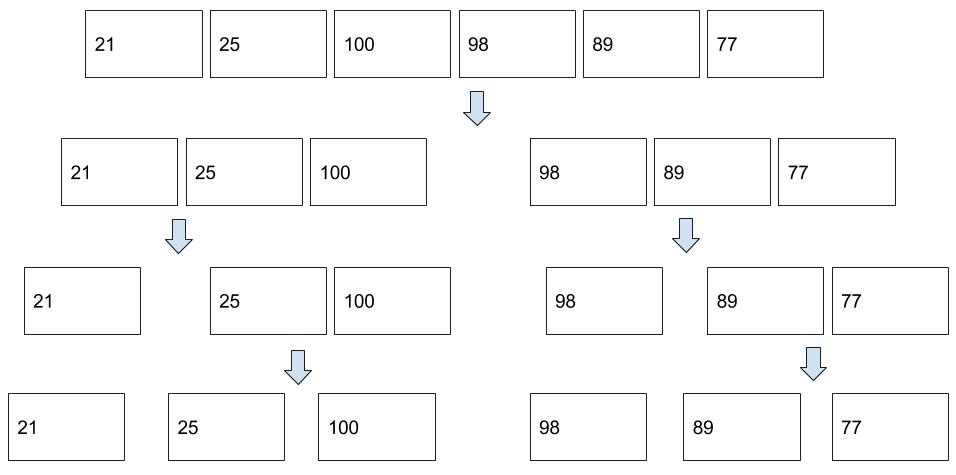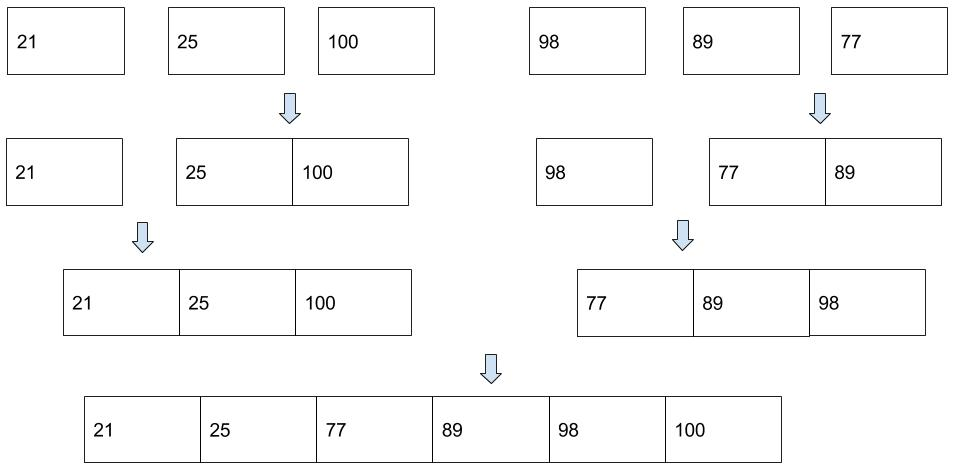### Data Structure and Algorithm

In this tutorial, we will learn a simple sorting algorithm - Merge Sort.

## Table Of Content

###### 1. Problem to Solve

Given a list of numbers as shown below, please sort them in ascending order.

Requirements:

• You are required to use Merge Sort algorithm when sorting the numbers.
• You are required to implement the algorithm in PHP language.
###### 2. Pseudocode

Merge Sort is a divide and conquer algorithm. It works by continually splitting a list in half until both halves are sorted, then the operation merge is performed to combine two lists into one sorted new list.

When splitting a list, we consider the list is sorted if it contains zero or one element.

Split:Merge:Pseudocode of Merge Sort algorithm can be written as follows:

###### 3. PHP Implementation

As we can see, there are two procedures in this algorithm. This leads us to two PHP functions, where the first function(mergeSort) involves a recursion.

We have followed the pseudocode strictly to implement the algorithm in PHP. The only parts that we want to highlight are a couple of built-in PHP array functions:

• array_slice: It extracts a slice of the array. This function is handy when we want to a certain part of the array.
• array_shift: It remove an element from the beginning of an array. This function is handy when we want to remove the first element of the array.